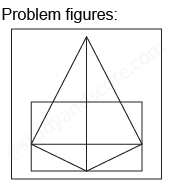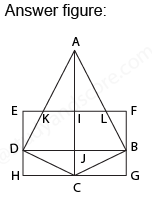## Practice Test Discussion

Q)

In each of the following question, find the minimum number of triangles in the given figure.• ExplanationAfter labelling the figure, we get the following,

• Simplest triangles AKI, AIL, EKD, LFB, DJC, BJC, DHC and BCG (8)
• Triangles with two components each AKL, ADJ, AJB and DBC (4)
• Triangles with three components each ADC and ABC (2)
• Triangle with four components is ABD (1)

Total number of triangles in the figure is 8 + 4 + 2 + 1 =15

Hence option A is correct.

- Share with your friends! -